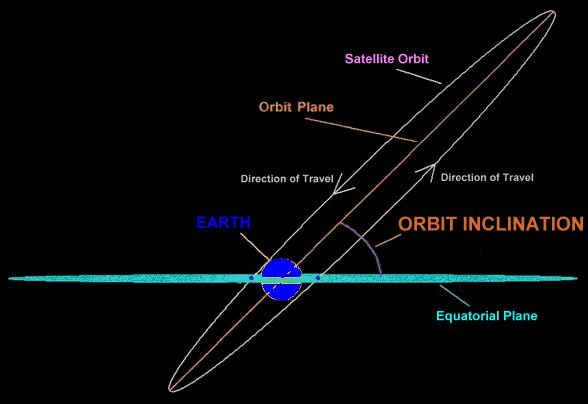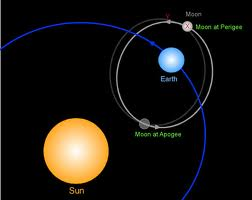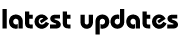IAS Mantra Series : Satellite Communication -4

Q1.   Define the terms (i) orbit path (ii) orbit radius (iii) orbit plane (iv) prograde orbit (v) retrograde orbit

Ans.: Orbit path

The path followed by an orbit is circular.  An orbit of a satellite may be elliptical or circular  A geostationary satellite has circular orbit path.When a satellite moves in a circular orbit, the radius of the circle is called orbit radius.

Orbit plane

The plane in which a satellite orbits is called the orbit plane. A man-made satellite may lie in polar plane, equatorial plane or inclined plane.

When an artificial satellite orbits in the same direction of revolution of earth, the orbit is called prograde orbit. Satellites are usually launched in prograde orbits.When an artificial satellite orbits in the opposite direction as the direction of revolution of earth, the orbit is called retrograde orbit.

Q2.   What is the difference between a geosynchronous satellite and a geostationary satellite?

Ans.:   A satellite which moves in the orbit path with the same angular velocity as that of revolution of earth around its axis of rotation is called geosynchronous satellite.

A satellite which is geosynchronous in the equatorial plane and has pro-grade circular orbit is called a geostationary satellite.

The orbit period of a geosynchronous satellite as well as a geostationary satellite is 24 hours.

A geostationary satellite always appear to be stationary by an observer on the surface of the earth.

Q3.   What is a direct satellite?

Ans.:   A satellite launched in the direction of the earth’s motion is called a direct satellite.

Q4.   What is a retrograde satellite?Ans.:   A satellite launched in the opposite direction to the earth’s motion is called a retrograde satellite.

Q5.   What is Clarke orbit?

Ans.:   The geosynchronous orbit of a satellite is also called a Clarke orbit.

Q6.   Name six important orbital elements of a satellite.

Ans.:   These are

1. Semi-major axis

2. Time of perigee

3. Right ascension of ascending node

4. Inclination

5. Argument of perigee

6. Eccentricity

Q7.   What is the first point of Aries?

Ans.:   The first point of Aries in a fixed location in space. It is the direction of a line from the centre of the earth through the centre of the sun at the vernal equinox i.e. about 21st March.

Q8.   What are the various factors, which cause orbital perturbations of an artificial satellite?

Ans.:   The following factors cause orbital perturbation of an artificial satellite.

1. Gravitational fields of the sun and the moon

2. Solar and lunar eclipses

4. Atmospheric drag (for a low-earth orbit satellite)

5. Asymmetric gravitational field due to earth’s oblateness.

Q9.   What are the effects of orbital perturbations of a satellite?

Ans.:   Due to orbital perturbations the true orbit of the satellite does not remain a Keplerian ellipse. The satellite does not return to the same point in space per revolution. As a result, the sub-satellite point of a geo-synchronous satellite moves with time. Due to these the longitude and inclination of a satellite may change.

Q10.   What is the anomalistic period?Ans.:   Orbital perturbations lead to anomaly in the period of revolution of a satellite round the earth. The time of elapse between successive perigee passages of a satellite under orbital perturbations is called the anomalistic period.

Q11.   What are the reasons of orbital perturbations due to earth’s oblateness?

Ans.:       The earth is a triaxial ellipsoid. It is flattened at the poles. The equatorial radius is also not constant. Besides, there are regions where the average density of the earth appears to be higher. These regions of mass concentrations are called mascons. Due to these three factors i.e. the non sphericity of the earth, the non-circularity of the equatorial radius and the Mascons, there is non-uniform gravitational field around the earth. Therefore the force on an orbital satellite will vary with position. These effects tend to change the longitude of the satellite in space.My Great Web page